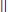NextPrevious

# How is the magnitude of a vector determined?

The magnitude of a vector is equivalent to the length of a vector. Placing a pair of vertical lines (similar to the absolute value symbol) around a vector implies the magnitude of the vector. For example, if the variable V is used to represent a vector, then the expressionindicates the magnitude of the vector.

Close

This is a web preview of the "The Handy Math Answer Book" app. Many features only work on your mobile device. If you like what you see, we hope you will consider buying. Get the App